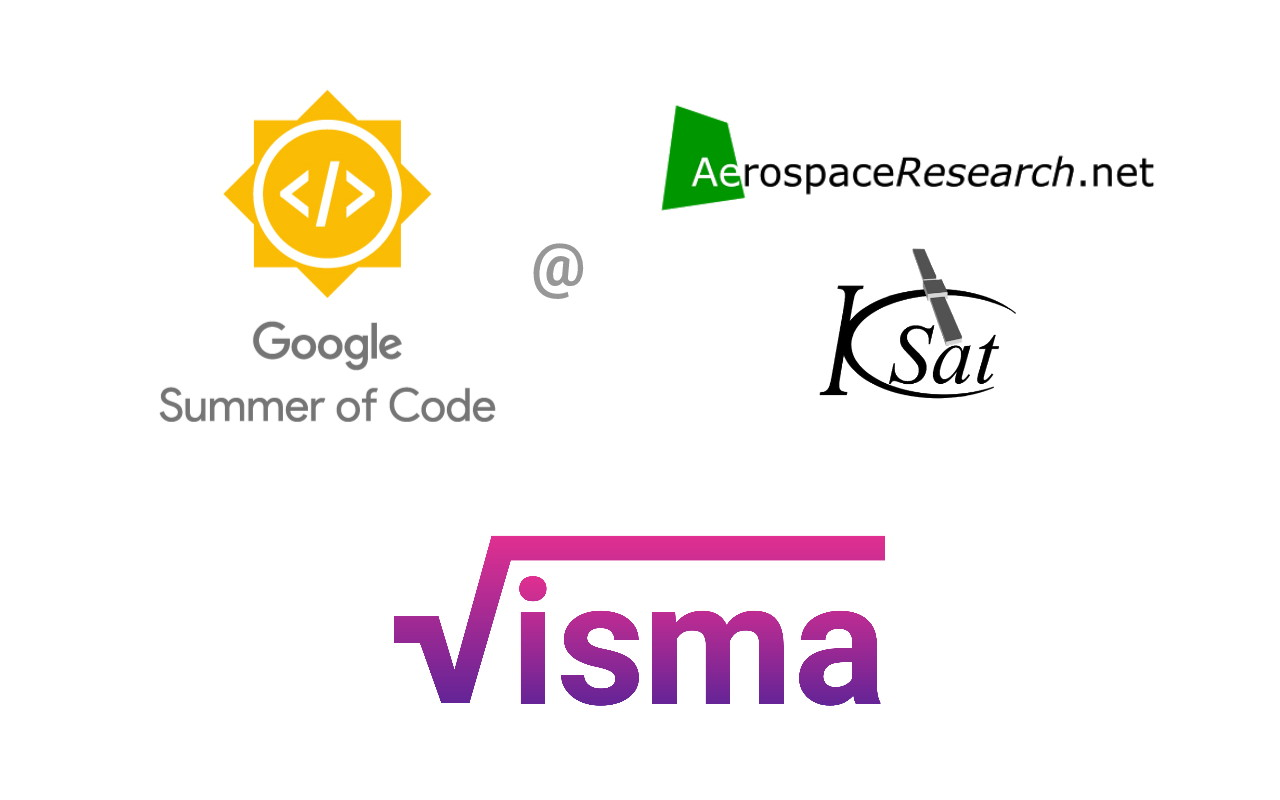# [GSoC’18|VisMa|Shantanu] Week #10-11: Dynamic Simplification and Plotting

This is GSoC’18 log#05. Here I will cover on what I have done in week #10-11. Link to the previous log. The work during this period is mostly done in visma/gui.## Done so far…

A quick solver (visma/gui/qsolver.py) has been implemented which dynamically simplifies the expression as the user inputs. Instead of implementing the logger which was supposed to report if the input expression is invalid, I have implemented this feature in the qsolver itself. It lets the user know what is missing in the input or if the input syntax is wrong.

A basic plotter using matplotlib was implemented in the first phase which plotted the result in 2D. It is now modified for plotting graphs in both 2D and 3D. The 3D graph is a mesh which resembles the surface of the input. Given the input, plotter decides (based on the number of variables and the type of input) in which dimension is the graph to be plotted.

The plotter logic is:

```if (noOfVars == 1 and eqType == "expression") or (noOfVars == 2 and eqType == "equation"):
graphVars, func = plotIn2D(LHStok, RHStok, variables)
elif (noOfVars == 2 and eqType == "expression") or (noOfVars == 3 and eqType == "equation"):
graphVars, func = plotIn3D(LHStok, RHStok, variables)```

The above snippet can be found here.

For example:

• If input is x^2 , the plot f(x) = x^2 is plotted in 2D.
• If input is x^2 + y^2 = 5 , the plot x^2 + y^2 – 5 = 0 is plotted in 2D.
• If input is x^2 – 2*y , the plot x^2 – 2y = 0 is plotted in 3D. This case (expression type with 2 variables) needs a fix. A plot for f(x,y) = x^2 – 2y must be plotted instead.
• If input is x^2/2 + y^2/5 = z , the plot 0.5x^2 + 0.2y^2/5 – z = 0 is plotted in 3D.

Below the qsolver and plotter can be seen in action.## What I will be doing next…

Almost all of the basic work related to GUI is finished. I will try to enhance the UI by adding settings menu and options to enable or disable certain UI elements. A simple option for enabling/disabling qsolver has been already added.

In the coming days, I will package visma for all desktop operating systems(Linux, Mac, and Windows). I am looking into pyinstaller for packaging visma for windows. Also in the final weeks, I will write docstrings and inline comments for the code, and update the github wiki.

Link to project source and to-do board.